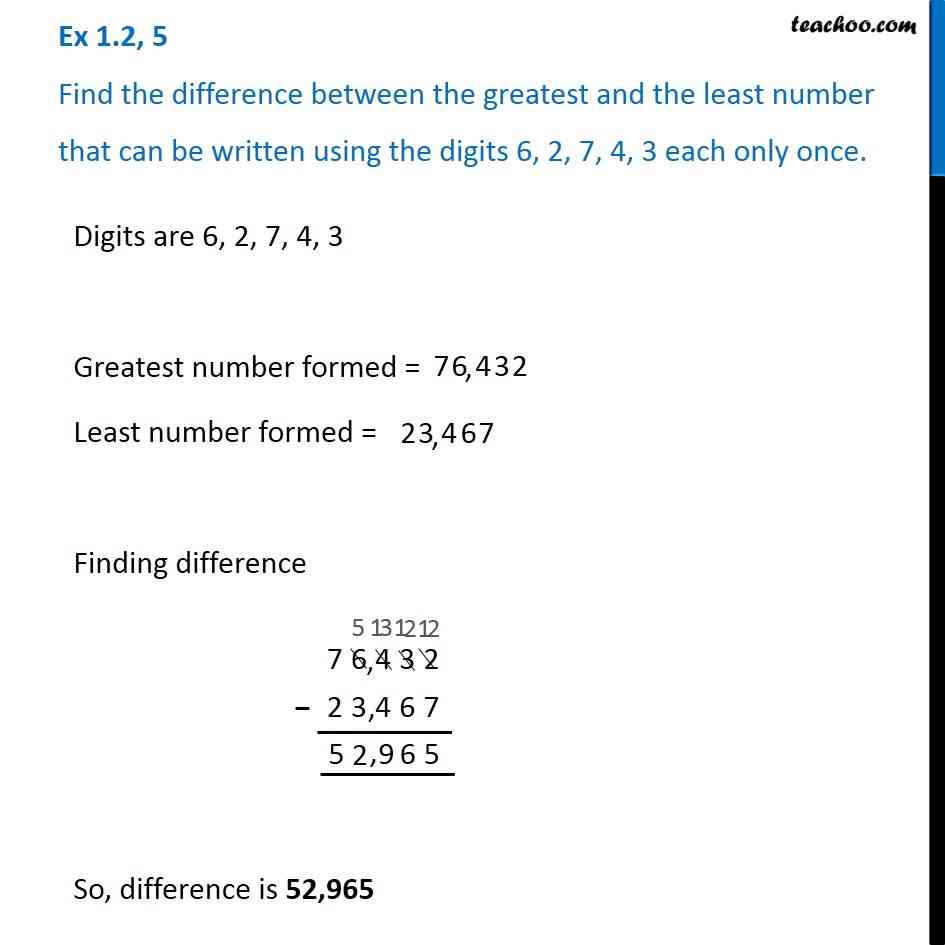1. Chapter 1 Class 6 Knowing our Numbers
2. Concept wise
3. Subtraction of large numbers

Transcript

Ex 1.2, 5 Find the difference between the greatest and the least number that can be written using the digits 6, 2, 7, 4, 3 each only once.Digits are 6, 2, 7, 4, 3 Greatest number formed = Least number formed = Finding difference So, difference is 52,965

Subtraction of large numbers